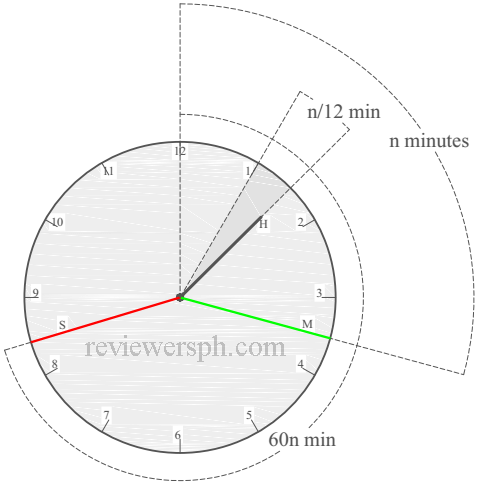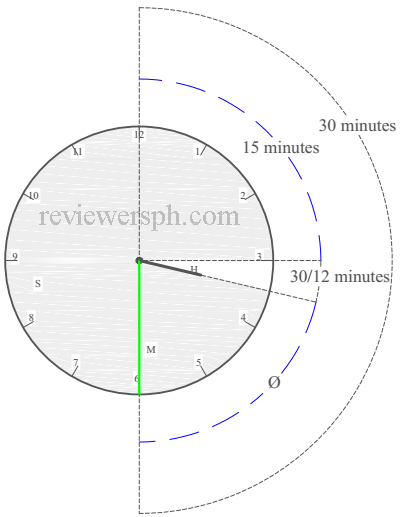### Math Notes

Subjects

#### Algebra Solutions

##### Topics || Problems

Calculation of Clock Problems

In clock problems you usually calculate time and angles.

Clock Hands

• Minute Hand (Base)
• Hour Hand
• Seconds Hand
As described by the minute hand
Minute Hand Second Hand Minute Hand Hour Hand
1 min 60 min 1 min 1/12 min
2 min 120 min 2 min 1/6 min
30 min 1800 min 30 min 5/2 min
... ... ... ...
n min 60n min n min n/12 min

This simply means that, if the minute hand moves n minutes the second hand moves 60n minutes and the hour hand moves n/12 minutes.Conversion factor between minutes to degrees
Minutes Angle (degree) from 12
15 90
30 180
60 360

Use this as a conversion unit, so if you like to calculate how many degrees is 12 minutes. 12 x 90 / 15 = 72 degrees

What is the angle between the hour hand and the minute hand at 3:30?

Solution:

sum forward (blue line) = $$15 + 30/12 + \theta$$

sum backwards (black line) = 30

$$15 +30/12 +\theta$$ = 30

$$\theta$$ = 25 minutes = 75 degreesUsing the hour hand, rewrite it as described by minute hand. From 12 to 3 that's 15 minutes, from 3 to where it is now is 30/12 minutes(refer to the table above) and the rest up to 6 is unknown.

The minute hand just moves 30 minutes.

Another Solution:

Convert all minutes to degrees

15 minutes = 90 degrees

30/12 minutes = 15 degrees

30 minutes = 180 degrees

sum forward (blue line) = $$90 + 15 + \theta$$

sum backwards (black line) = 180

$$90 + 15 + \theta$$ = 180

$$\theta$$ = 75 degrees